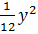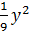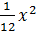### Sample Problem

What is the equation of a parabola with vertex at the origin and focus at (3,0)?

(A) x =(B) x =(C) y =(D) y =#### Solution

4xp = y2

4(3)x = y2

12x = y2

x =